Next: Discussion Up: Synchronous Oscillations Based on Previous: Modeling Cortical Oscillations

# Why Local Connections?

Temporal correlation promises to provide a conceptual framework for scene segmentation and figure/ground segregation [4,42,43,57,62,64,63], a tremendous problem facing current technology of general pattern recognition . However, there is an outstanding obstacle to this idea if synchrony can only be produced with full connections as in associative memory models or with a global phase coordinator (they are computationally equivalent). A network with full connections is dimensionless, and loses the critical information of geometry. Thus it would lead to indiscriminate synchronization among all object features, contrary to Gestalt laws of perceptual grouping that emphasizes spatial and temporal relationships of the objects [32,46]. This point can be clearly illustrated in Figure 6a, where two objects, a desk lamp and a cup, are placed on a two-dimensional 15x15 grid. One can easily segment them solely on the basis of connectedness, which turns out to be one of the most important Gestalt grouping principles . However, if the oscillators on the grid are fully connected, there would be no way to segment the two, since an active oscillator, say, triggered by the cup image, projects indiscriminately to the other oscillators activated by the cup as well as the ones activated by the desk lamp. This is the case even we take into account the orientation sensitivity, so that only the oscillators triggered by the same orientation are fully connected as used in [4,55].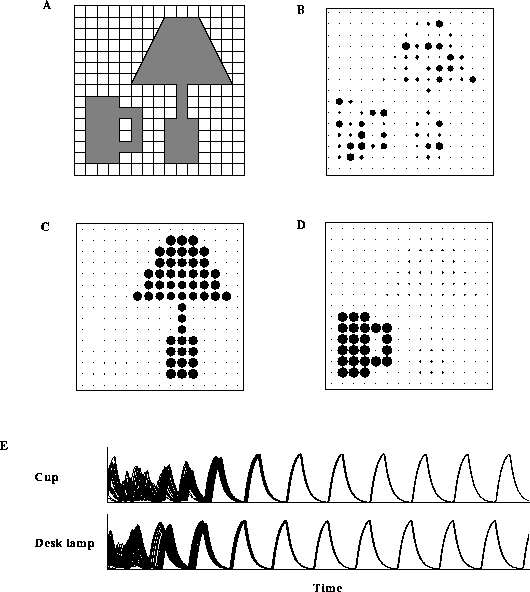Figure 6: Scene segmentation based on local coupling of neural oscillators. ( a) The two images, representing a ``cup'' and a ``desk lamp'', are presented to a 15x15 matrix of oscillators with nearest neighbor connections (See also ). ( b) A snapshot of the activities of the oscillator grid at the beginning of dynamic evolution. ( c) A snapshot of the activities of the oscillator matrix several cycles after the beginning. ( d) Another snapshot taken shortly after (C). In these displays, the x activity of an oscillator is denoted by the radius of the corresponding circle, and only the oscillators with nonzero activity are shown. ( e) The upper trace shows the combined temporal activities of the oscillators representing the cup image, and the lower trace shows those representing the desk lamp image. The height of each ordinate is 1. Stimulated oscillators received external input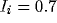, and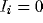for the remaining ones. The initial values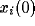and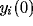were randomly generated within the range [ 0.8,0]. The parameters for a single oscillator are the same as in Figure 2 except that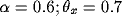; The overall incoming connection strength for each oscillator was normalized to 1.5. In this simulation, 7,000 integration steps were used.

We believe that the mechanism elucidated here provides a way out of this predicament. Sensory segmentation can now be accomplished based on general architectures with connections only in neighboring units. The two-dimensional matrix of Figure 5 can readily serve for segmentation based on connectedness and proximity, known to be essential for perceptual grouping. Let us demonstrate it by solving the segmentation problem of Figure 6a. Each cross point in the grid is represented by an oscillator defined in the Model Description ... section, and each oscillator is only connected to its four nearest neighbors except boundary ones where we assume no wrap-around. The objects are presented to the model simply by setting the inputs to the oscillators covered by the objects to a high value. Thus, the stimulated oscillators are activated, while the others are inactive. The phases of the activated oscillators were initially randomized. With the dynamic links in place, the oscillators belonging to the same connected region are quickly entrained. That is, the oscillators constituting the cup image are synchronized, and the same is true for those constituting the desk lamp image. But since there is no interconnections between the two objects, their oscillations are not correlated. The rest of Figure 6 shows the simulation results. Figure 6b shows a snapshot (instant activity) of the oscillator network shortly after the images were applied to the network. As is clear from the figure, the phases of the oscillators were fairly random. But, just a few cycles later, the oscillators representing each object were synchronized, which can be seen by the same amplitudes of oscillations exhibited by those oscillators. Figure 6c and d show two other snapshots after synchrony across each image was established, where in Figure 6c the desk lamp image dominates and in Figure 6d the cup dominates. The entire temporal process of reaching synchrony within each object is shown in Figure 6e, where the two traces represent the combined oscillatory activities of the oscillators belonging to the two objects respectively. The synchronized oscillations within each object are clearly shown just a few cycles after the beginning of dynamic evolution.

In the above simulation, no attempt was made to desynchronize the oscillators belonging to different objects, which should be included in a full system of image segmentation. We can achieve desynchronization by introducing a global inhibitor that receives inputs from the entire network and inhibits back. Equivalently, the global inhibitor can be replaced by long-range weak mutual inhibitions (see Wang et al. , for such an example). Desynchronization among different patterns will be discussed in the postscript.Next: Discussion Up: Synchronous Oscillations Based on Previous: Modeling Cortical Oscillations